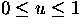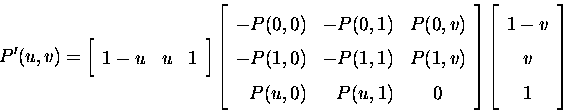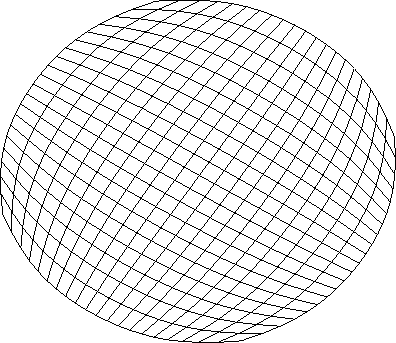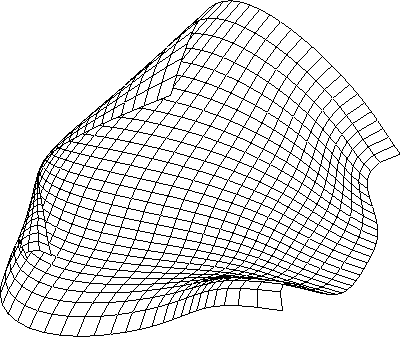Next: Algorithm and implementation Up: Surface Triangulation Previous: Surface Triangulation

# Input : Linear Coons surface

The input for the surface triangulation routine implemented here is the bilinear Coons surface/patch [GH73,RA90].

If the four boundary curves in (u,v) space, P(u,0), P(u,1), P(0,v) and P(1,v) are known, and a bilinear blending function is used for the interior of the surface patch, a linear Coons surface is obtained. It is given by

where,. It can be easily seen that, at the corners,

P'(0,0) = P(0,0), P'(0,1)=P(0,1), P'(1,1)=P(1,1), P'(1,0)=P(1,0)

and along the boundaries,

P'(u,0) = P(u,0), P'(u,1)=P(u,1), P'(0,v)=P(0,v), P'(1,v)=P(1,v)

More compactly, the equation can written asA mapping function then gives (x,y,z) corresponding to every (u,v). In the implementation here, the four boundary curves happen to be B-spline curves (BSCs). The functions (1-u), u, (1-v), and v are called blending functions because they blend the boundary curves to produce the internal shape of the surface. The linear Coons surface is the simplest of the Coons surfaces. Examples of these surfaces are Figures 6.1 and 6.2. A C code for IITZeus using X/Motif was written as a part of this project to obtain this.Next: Algorithm and implementation Up: Surface Triangulation Previous: Surface Triangulation
Anirudh Modi
1/16/1998